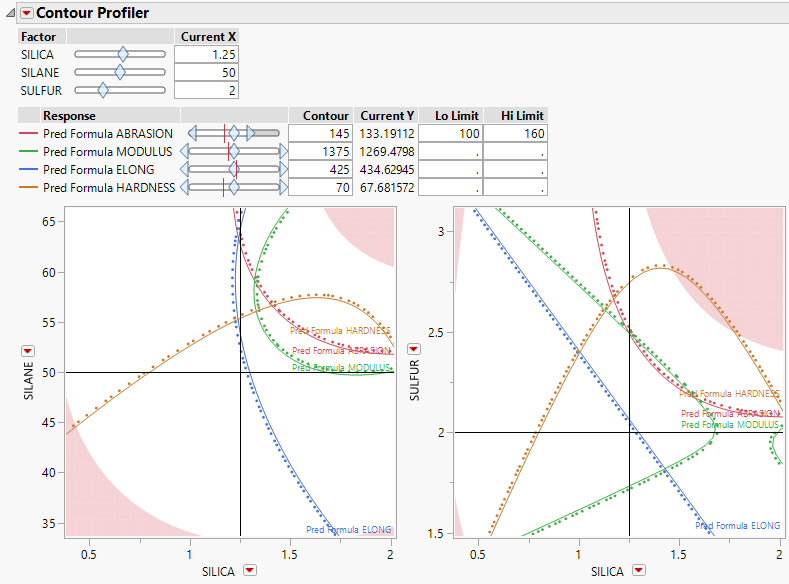Profilers > Contour Profiler > Example of the Contour Profiler
Publication date: 07/30/2020

# Example of the Contour Profiler

This example uses the Tiretread.jmp sample data table. There are three factors (SILICA, SILANE, and SULFUR) and four responses (ABRASION, MODULUS, ELONG, and HARDNESS). This data table already contains saved prediction formula columns for the four response variables.

1. Select Help > Sample Data Library and open Tiretread.jmp.

2. Select Graph > Contour Profiler.

3. Select Pred Formula ABRASION, Pred Formula MODULUS, Pred Formula ELONG, and Pred Formula HARDNESS and click Y, Prediction Formula.

4. Click OK.

5. Click the Contour Profiler red triangle and select Multiple Contour Frames.

6. Under Horizontal Factor, select SILICA and under Vertical Factor, select SULFUR.

7. Click OK.

On both contour plots, the values for SILICA are on the horizontal axis. The values for SILANE and SULFUR are on the vertical axes. In the first contour plot, the value for SULFUR is fixed at 2.25. In the second contour plot, the value for SILANE is fixed at 50 (Figure 4.2).

8. Click the Current X box for SULFUR and type 2. Click anywhere outside the box to set the value.

9. In the Lo Limit box for Pred Formula ABRASION, type 100.

10. In the Hi Limit box for Pred Formula ABRASION, type 160.

11. Click anywhere outside the box to update the plot.

Figure 4.3 Contour Profiler for Tiretread.jmpThe contour lines in the first contour plot differ from those in Figure 4.2 because SULFUR is set at a different value. This can be seen in the placement of the horizontal line at 2 in the second contour plot. Move the slider bar for SULFUR to see how different values further change the contour lines in the first contour plot. Move the slider bar for SILANE to see how different values change the contour lines in the second contour plot. The shaded area on the contour plots represent the regions of the graphs that are not feasible, based on the Lo Limit and Hi Limit values for ABRASION.